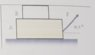# Forces acting on a block which is lying on another block

• Like Tony Stark
Like Tony Stark
Homework Statement
In the picture, the coefficient of static and dynamic friction for all contact surfaces are ##0.4## and ##0.14##, respectively. The mass of ##A## is ##73 kg## and the mass of ##B## is ##22 kg##. Determine the maximum force so that B doesn't slide. Then, suppose that the force applied to the system is greater than the force calculated previously, how much time would it take for the blocks to move ##10 m##?
Relevant Equations
Newton's equations
Well, I'm having trouble with the free body diagrams. For ##A## we have
##y)## weight, normal force, contact force with ##B##, ##F . sin(36.8°)##. And the acceleration is ##0## because we want to calculate the maximun force before moving.

##\Sigma \vec F = m . a_y##
##\vec N_A + \vec F . sin(36.8°) - \vec W_A -\vec Fc= 0##

##x)## ##F . cos(36.8°)##, friction force with the ground and friction force with ##B## and the acceleration is 0.

##F. cos(36.8°) - Fr - Fr_B=0##

##Fr## and ##Fr_B## have the same direction, don't they? Because the friction with ##B## must be pushing ##B## towards, and then the reaction force is in the opposite direction.

For ##B## we have:
##y)## weight and contact force (normal force)

##\vec N_B - \vec W_B =0##

##x)## friction force with ##A##
But is this force pointing to the right? And then, is the string applying a force on ##B##?

And then when I want to answer the second question, do they move equally? I mean, if ##A## traveled ##10 m## so did ##B##

#### Attachments

•20190927_085748.jpg
31.6 KB · Views: 134

Homework Helper
Gold Member
2022 Award
normal force, contact force with B
The contact force with B could include a frictional component in general. Better to say normal force from ground, normal force from B.
the acceleration is 0 because we want to calculate the maximun force before moving.
No, it's the maximum force before B slides on A. A will be moving by then.

Like Tony Stark
The contact force with B could include a frictional component in general. Better to say normal force from ground, normal force from B.

No, it's the maximum force before B slides on A. A will be moving by then.

So the free body diagram for A is
##x) F.cos(36,8°)-Fr-Fr_{AB}=m.a_x##
##y) F.sin(36,8°)+N-P-F_{AB}=m.a_y##

And for B is
##x) Fr_{AB}=m.a_x##
##y) F_{AB}-P=m.a_y##

Is this correct?

And for the ##y)## axis of both bodies the acceleration is equal to 0, isn't it? Because they don't move upwards. Is this right?

Homework Helper
Gold Member
2022 Award
Is this correct?
Yes, except what is P in these equations? Not the same in both, I assume.
And for the y) axis of both bodies the acceleration is equal to 0
Yes.

Like Tony Stark
Yes, except what is P in these equations? Not the same in both, I assume.

Yes.
Sorry, ##P## is weight
Thanks!

Homework Helper
Gold Member
2022 Award
Sorry, ##P## is weight
Thanks!
Ok, but different weights for the two blocks, right?

Like Tony Stark
Ok, but different weights for the two blocks, right?
Yess